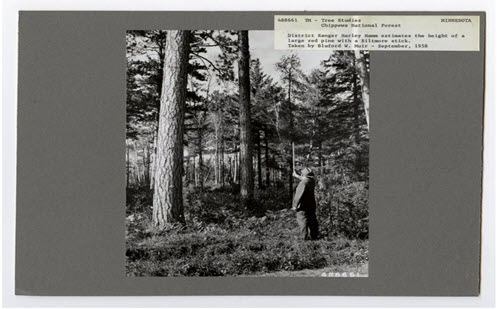Go to website

# reSolve: Tree Biomass

In this sequence of two lessons, students investigate how many trees would be required to supply paper for their school for a year. Students use similar triangles, Pythagoras' Theorem and algebra to design and construct a Biltmore stick, used to measure the diameter and height of a tree. They measure trees, calculate their volume and use the density of the tree to find the dry mass of the tree. They estimate the amount of paper used in the school and hence estimate the number of trees that need to be cut down to supply the school's paper needs. The lessons are outlined in detail including curriculum links, vocabulary, sample answers, discussion points and student resources. This sequence is part of the reSolve: Mathematics by Inquiry program.

Year level(s) Year 8, Year 9
Audience Teacher
Purpose Teaching resource
Teaching strategies and pedagogical approaches Mathematics investigation
Keywords volume, cylinders, ratios, scale, similarity

## Curriculum alignment

Curriculum connections Numeracy
Strand and focus Measurement, Build understanding, Apply understanding
Topics Area, volume and surface area, Proportion, rates and ratios, Pythagoras and trigonometry
AC: Mathematics (V9.0) content descriptions
AC9M8M06

Use Pythagoras' theorem to solve problems involving the side lengths of right-angled triangles

AC9M9M01
Solve problems involving the volume and surface area of right prisms and cylinders using appropriate units

AC9M9M03

Solve spatial problems, applying angle properties, scale, similarity, Pythagoras' theorem and trigonometry in right-angled triangles

Numeracy progression Understanding units of measurement (P9, P10)
Understanding geometric properties (P7)
Proportional thinking (P7)
Multiplicative strategies (P9)